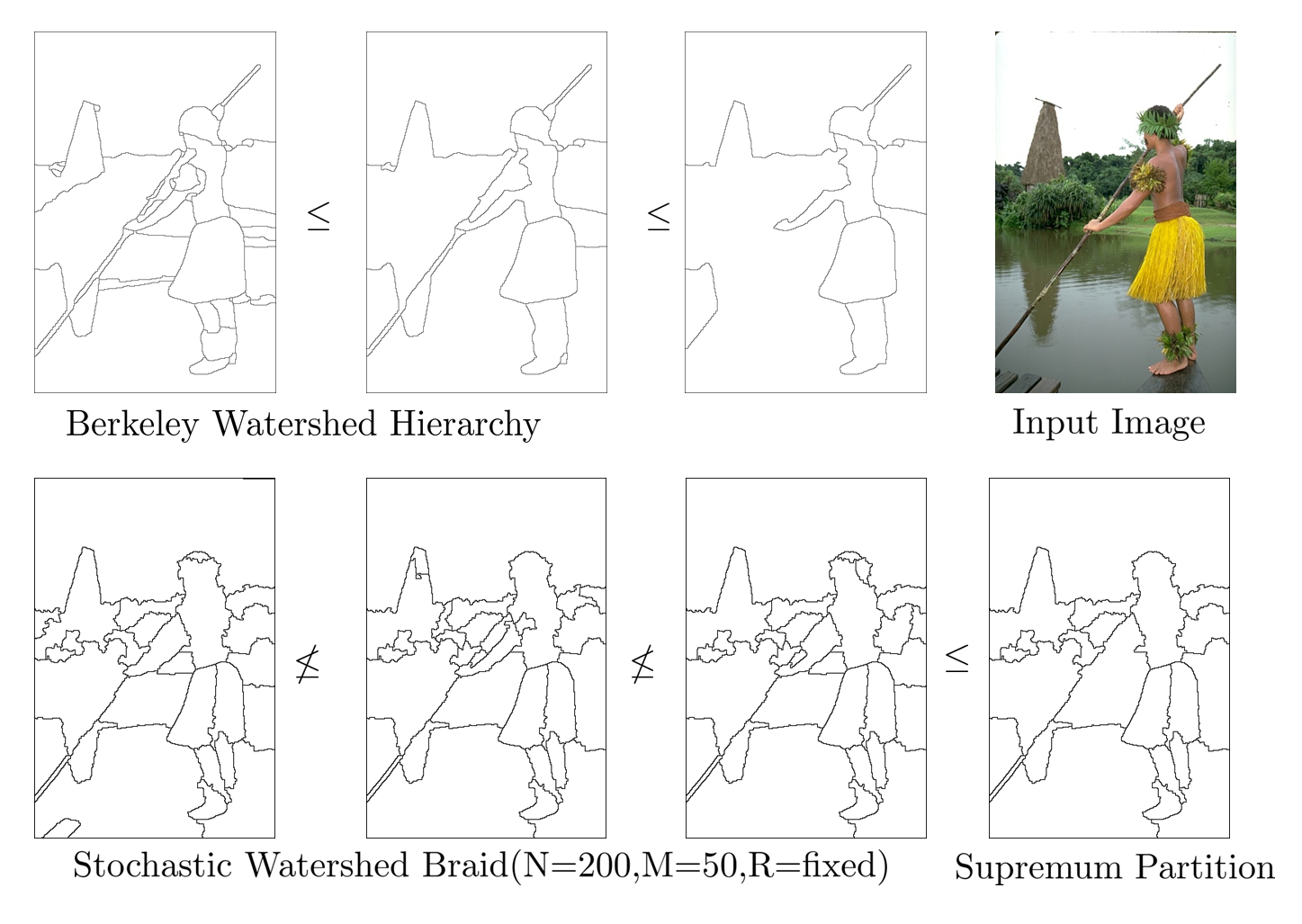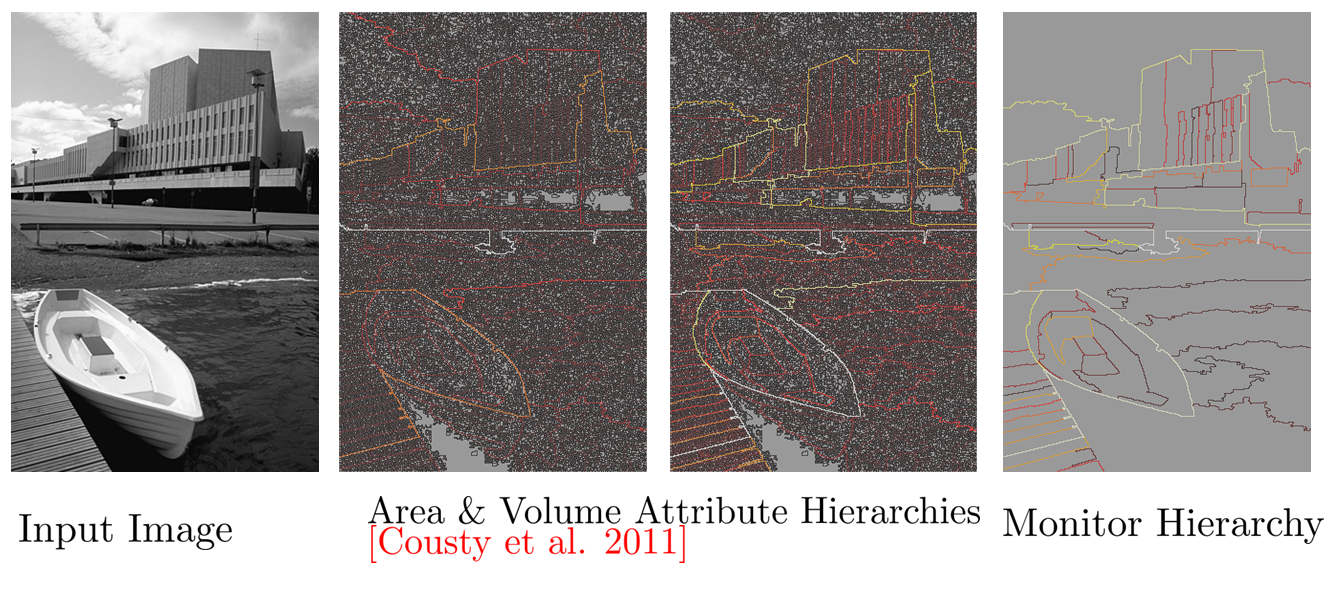# Cost-complexity pruning of random forests ISMM 2017

Given a hierarchy of partitions $$H = \{ \pi_i \}_{ 0 \leq i n}$$ the optimal cut problem aims at calculating :

\begin{aligned} & \underset{\pi \in \Pi(E,H)}{\text{minimize}} & & \sum_{S \in \pi} \omega(S)\\ \end{aligned}

The problem can be solved greedily using the dynamic program whose substructure is given by

$$\pi^{\ast}(S) = \begin{cases} \{S\}, & \text{if}\ \omega({S})\leq \sum(\omega(\pi^\ast(a))), a \in \pi(S)\\ \bigsqcup_{a \in \pi(S)} \pi^\ast(a), & \text{otherwise} \end{cases}$$

$$h$$-increasingness provides the condition on the energy defined on the partial partitions $$\omega(\pi(S))$$ to so as to obtain a global optimum :

$$\omega(\pi_1(S)) \leq \omega(\pi_2(S)) \implies \omega(\pi_1(S) \sqcup \pi_0) \leq \omega(\pi_2(S) \sqcup \pi_0)$$

There can be many solutions to the dynamic program. We impose uniqueness by imposing the following condition on $$\omega$$ :

$$\forall \ \pi(S)\in\Pi(S), \ \ \omega(\{S\})\neq\omega(\pi(S))\}$$

Given the uniqueness condition the energetic-ordering between two partitions $$\pi_1, \pi_2$$ is :

$$\pi \preceq _{\omega }\pi^\prime \ \Leftrightarrow \ \forall S\in \pi \vee \pi^\prime \ \text{we have} \ \omega (\pi \sqcap \{S\})\leq \ \omega(\pi^\prime \sqcap \{S\})$$

Here $$\pi \preceq _{\omega } \pi^{\prime}$$In fact during the optimization procedure one never evaluates energy of a partition, but only of the partial partitions. This is the cruicial part of the problem which renders the dynamic program to be forumlated as a lattice structure. The energetic lattice ( $$\preceq _{\omega }, \vee_{\omega}$$ ) derives from the energetic order.The hierarchy of partitions can be created using two different types of operations. Given a elementary leaves partition one can create the hierarchy bottom-up by fusing classes of the partitions together creating coarser parent partitions (child->parent fils -> pere). Or given a coarse elementary partition one can create the hierarchy top-down by dividing classes of the partitions into finer children classes (parent->child pere -> fils).

While the braid of partitions consists of a third type of refinement operation, including the fusion and division operations. This is termed as hierarchical reorganization, while mathematically it corresponds to local partitioning that keeps the pairwise supremum partitions a hierarchy of partitions. More particularly, this corresponds to a fusion-division (or division-fusion) operation on the same set of classes in a partition, that produces a new local partitioning of the space. Braids are the largest family over which the dynamic programming substructure is preserved to yield a global optimum.

$B = \{\pi_i\}_{i=1}^n | \pi_i \vee \pi_j \in \Pi(E,H)$

We can see from the braid structure that it is in accordance with the energetic ordering and also generalizes hierarchical partitioning of the space while preserving the dynamic program's substructure.</p>

### Examples of partition families that form braids

The Ultrametric contour map(UCM) from the berkeley group is an example of a hierarchy. While the Stochastic watershed by Angulo et al. is an example of a braid. There is a total ordering between the partitions in a hierarchy, while there is a partial ordering for partitions belonging to a braid. The supremum partitions of a braid though turn out to be hierarchical. This provides a generalization of the hierarchical structure for the purpose of dynamic programming and search of better solution spaces.

#### Stochastic watershed Braids:#### Composition of Attribute watershed hierarchies:### Dynamic programming substructre on BraidsTo ensure that one reaches the minimum using previously calculated results over finer partial partitions, we use the hierarchical increasingness property. This ensures the monotonic property of the energy during the bottom-up/top-down scan by the dynamic program.

$$h$$-increasingness is over partial partitions from a hierarchy and a braid is demonstrated. We see that the braid provides a larger family of partitions over which the dynamic program works. This requires a partial ordering in case of braids.

• $$S \supset \pi(S)$$, fusion

• $$\pi(R) \subset R$$, division

• $$\pi(X) \vee \pi(X^\prime)$$, partial partitioning

Human annotated & algorithm segmentations match in three different ways (shown below)## Optimal Cuts from hierarchies of partitions

• Preprint: PR journal 2013

• Keywords : Hierarchical segmentation, MathematicalMorphology, Energy minimization, Dynamic programming

• Algorithms

• Optimal Cut Algorithm : Breimans algorithm to calculate the optimally pruned rooted tree.

• Optimal hierarchy using scale-sets : Complete hierarchy of optimal cuts by ordered by scale function.

• Scale-function is calculated by equating lagrangians of parent and child energies

#### Optimal Cut (Luminance) VS Optimal Cut (Chrominance)

• Original Image• Fidelity term (Luminance)$$\omega_{\text{Lum}}(S) = \sum_{x \in S} \|l(x)-\mu(S)\|^2 + \lambda(24 + |\partial S|)$$

• Fidelity term (Chrominance)$$\omega_{\text{Chrom}}(S) = \sum_{x \in S, i} \|c_i(x)-\bar{c}_i(S)\|^2 + \lambda(24 + |\partial S|)$$

• Texture Enhancement by including class size unformity term in Mumford Shah Functional

Mean size of siblings $$\mu_A = \frac{\sum_{S^\prime \in \text{siblings}(S)} |S|}{|\text{siblings}(S)|}$$

Texture energy $$\omega_{\text{texture}}(S) = \omega_{\text{Chrom}}(S) + \frac{\nu}{\sum_{S^\prime \in \text{siblings(S)}} |\mu_A-|S^\prime||^2}$$

### Intial Image - VS - For weak uniformity - VS - For strong uniformity:### Related Publications and slides

• Energetic Lattices and braids : Talk at Icube-MIV March 2017, Slides

• Braids Of partitions, B Ravi Kiran, Jean Serra, ISMM 2015.HALSlides, bibtex

• Constrained Optimization on Hierarchies and Braids, Jean Serra, B Ravi Kiran, ISMM 2015HALPoster

• Review on braids of partitions : Talk at CMM March 2015 Slides

• B. Ravi Kiran, Jean Serra, Global local optimizations by hierarchical cuts and climbing energies, In press, Pattern Recognition, Volume 47, Issue 1, January 2014, Pages 12–24. ISSN 0031-3203.

• Optima on Hierarchies of Partitions, J. Serra, B.R. Kiran, ISMM 2013, LNCS, Vol. 7883, pp 147-158

### Tutorial on optimization on hierarchies at ICIP 2014

• Jean Serra: Hierarchies and Energetic Lattice Slides

• Hugues Talbot: Optimisation in Imaging Slides

• B Ravi Kiran: Constrained Optimization on Hierarchies Slides

• Jean Cousty: Handling and computing hierarchies with graphs Slides

• Toward Objective Evaluation of Image Segmentation Algorithms, Unnikrishnan 2007 PAMI

• Segmentation as Maximum-Weight Independent Set, Brendel, Todorovic NIPS 2010

• Ordered Hypothesis Machines Zimmer, Hush, Porter JMIV 2012

• Grain Building Ordering, J. Serra ISMM 2011

• Learning to Merge: A New Tool for Interactive Mapping, Porter SPIE 2013

• Orders on Partial Partitions Based on Block Apportioning, Christian Ronse 2014

• Classification and regression trees. CRC press, 1984, Breiman et al.

• BPT, efficient representation for image segmentation, Image Proc., Transactions 2000, Salembier et al.

• Guigues et al. “Scale-sets image analysis.” IJCV 2006

• GloBo : un algorithme stochastique pour l'apprentissage supervisé et non-supervisé pdf VOLATA

• Convex optimization, Stephen Boyd and Lieven Vandenberghe (2004)

• Level lines selection with variational models for segmentation and encoding. Ballester, C., et al. 2007

• Contour detection and hierarchical image segmentation, Arbelaez, P., et al. 2011

• Supervised assessment of segmentation hierarchies. Pont-Tuset, J. and Marques, F. (2012)

• Fast approximate energy minimization via graph cuts, Boykov, Y., Veksler, O., and Zabih, R. (2001)

• Some links between min-cuts, optimal spanning forests and watersheds, Allene, C., et al. 2007

• Watershed cuts: Thinnings, shortest path forests, and topological watersheds. Cousty. et al. 2010

• Power watersheds: A unifying graph-based optimization framework, Couprie, C., et al .2011

## Ground truth energies and Class opening

During the evaluation of ground truth segmentation one problem is the evauation of a numerical measure that determines if the given image segmentation is close to the expert/human drawn ground truth. Given a hierarchy of segmentations one faces the problem of chosing which partition amongst all cuts is closest to a given ground truth. We evaluate a localized haussdorf distance locally for every super-pixel segment belonging to the input hierarchy of segmentations corresponding to the input image, using the ground truth partition contours and their distance functions.First energy : Maximum distance function value of the contours of the segment over the contour of the ground truth passing within the segment, Second energy : Maximum distance function value of the ground truth contour over the segment contours.

These local energies termed as ground truth energies. These functions are h-increasing. Once, these energies calculated for every segment in the hierarchy, we can extract the partition whose with least value of composition of ground truth energies. The optimal cut extracted here thus corresponds to the partition from the hierarchy of partitions closest locally w.r.t each class, to the input ground truth partition.

• Net opening and applications to GIS Function is defined on the contours while segment/regions are zero valued. Thus a numerical function on the finest partition in fact defines a hierarchy of partitions.• (left) L-opening: corner based opening that removes L configurations from k-d-trees to transform then into valid quad-tree partitions (right) Contour curvature weighted net openings• Commune accessibility using distance maps of primary autoroutes in PACA -TBD## Related Publications

• Scale Space Operators on Hierarchies of Segmentations B Ravi Kiran, Jean Serra &nbsp; SSVM 2013,PDF

• Ground truth energies for hierarchies of segmentations B. Ravi Kiran, Jean Serra, ISMM 2013 PDF

• Fusions of Ground Truths and of Hierarchies of Segmentations, B Ravi Kiran, Jean Serra, Pattern Recognition Letters 2014.Link Slides

## References

• Bottom-up Segmentation for Top-down Detection

• Hierarchical Multi-Scale Supervoxel Matching using Random Forests for Automatic Semi-Dense Abdominal Image Registration